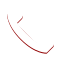Neural Networks & AI 2020-04-15T06:33:58+00:00

# Neural Networks & AI

Get familiar with Neural Networks & AI.

INR 60,000 + Taxes

# Neural Networks & AI

A neural network is a series of algorithms that actions to recognize fundamental relationships in a set of data through a progression that imitates the way the human brain functions. A neural network is a network or circuit of neurons, or in a contemporary sense, an artificial neural network, composed of artificial neurons or nodes. Neural networks can become accustomed to changing input; so the network generates the best imaginable result without necessitating to redesigning the output criteria.

Thus a neural network is either a natural biological neural network, made up of real biological neurons, or an artificial neural network, for solving artificial intelligence (AI) problems. These artificial networks may possibly be used for predictive modeling, adaptive control and applications where they can be taught via a dataset. Self-learning resulting from understanding can transpire within networks, which can develop conclusions or assumptions from a multifaceted and superficially distinct set of information.

Neural networks were first proposed in 1944 by Warren McCullough and Walter Pitts, two University of Chicago researchers who moved to MIT in 1952 as founding members of what’s sometimes called the first cognitive science department. Neural nets were a most important area of research in both neuroscience and computer science untiln1969, when, according to computer science lore, they were killed off by the MIT mathematicians Marvin Minsky and Seymour Papert, who a year later would become co-directors of the new MIT Artificial Intelligence Laboratory. The technique then enjoyed resurgence in the 1980s, fell into eclipse again in the first decade of the new century, and has returned with robust speed in the second, powered largely by the increased processing power of graphics chips.

At Integrum Litera we begin with training in detail about Introduction to Neural Net & AI, overview of Neural Net & AI, applications of Neural Net & AI, skills required for Neural Net & AI, steps involved in Neural Net & AI project, descriptive and inferential statistics, basic machine learning algorithms, advanced machine learning algorithms, time series analysis and forecasting, statistical analysis tools (R,Python & SAS), deep learning, neural networks, Image & text processing & lab sessions led by professionals, hands on real time projects, exclusive placement assistance followed by mock interviews and certification.

## CURRICULUM

#### Module 01: Statistics in Data science

Module 01: Statistics in Data science:

• Introduction to Data:
• Data types
• Data Collection Techniques
• Descriptive Statistics:
• Measures of Central Tendency
• Measures of Dispersion Measures of Skewness and Kurtosis
• Visualization
• Inferential Statistics:
• Sampling variability and Central Limit Theorem
• Confidence Interval for Mean Hypothesis Testing, t- Test, F-Test, Chi-square Test
• ANOVA
• Random Sampling and Probability Distribution:
• Probability and Limitations, Discrete Probability, Continuous Probability
• Bernoulli, Binomial Poisson distribution and Normal Distribution

#### Module 02: Association Rules Mining and Times Series Analysis

Module 2: Association Rules Mining and Times Series Analysis

1. Association Rules Mining:

• Apriori/Support/Confidence/Lift

2. Time Series Analysis:

• What is Times Series Data?
• Stationarity in Time Series Data
• Augmented Dickey Fuller Test
• The Box-Jenkins Approach
• The AR Process
• The MA Process
• What is ARIMA?
• ACF,
• PACF and IACF plots
• Decomposition of Times Series
• Trend, Seasonality and Cyclic
• Exponential Smoothing
• EWMA

#### Module 03: Neural Networks, Deep Learning & Practical Issues

Module 3: Neural Networks, Deep Learning & Practical Issues:

Introduction to Neural Networks and Deep Learning:

• Units/Neurons
• Weights/Parameters/Connections
• Single Layer Perceptron
• Multilayer Perceptron

Activation Functions:
• Sigmoid
• Tanh
• ReLU
• Leaky ReLU
• Convolutional Neural Networks
• Recurrent Neural Networks
• Image Processing
• Natural Language Processing

#### What is Neural Network?

Neural Network is a progression of algorithms that attempts to perceive underlying relationships in a set of data through a process that impersonates the way the human brain operates.vvv

#### What is an Artificial Neural Network(ANN)?

Artificial neural networks are one of the main tools used in AI. Neural is a brain-inspired system that is intended to reproduce the way that we humans learn.

#### What are the Elements of ANN?

Basic elements of ANN as follows;

• Processing Elements
• Topology
• Learning AlgorithmCall Now